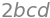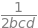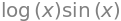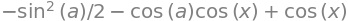# Solutions Q11 - 16#

# import all python add-ons etc that will be needed later on
%matplotlib inline
import numpy as np
import matplotlib.pyplot as plt
from sympy import *
init_printing()                      # allows printing of SymPy results in typeset maths format
plt.rcParams.update({'font.size': 14})  # set font size for plots


The first product $$\displaystyle \pmb{AB}=\begin{bmatrix} 19 & 22\\ 43 & 50 \end{bmatrix}$$ and the second $$\displaystyle \pmb{BA}=\begin{bmatrix} 23 & 34\\ 31 & 46 \end{bmatrix}$$, and as $$\pmb{AB} \ne \pmb{BA}$$ the matrices do not commute. The commutator $$[\pmb{A},\pmb{B}]=\pmb{AB}-\pmb{BA}$$ is obtained by subtraction and is the matrix $$\displaystyle [\pmb{A},\pmb{B}]=\begin{bmatrix} -4 & -12\\ 12 & 4 \end{bmatrix}$$.

$$\displaystyle \pmb{A}^2 = \begin{bmatrix} 1 & 1\\ 0 & 1 \end{bmatrix}\begin{bmatrix} 1 & 1\\ 0 & 1 \end{bmatrix}=\begin{bmatrix} 1 & 2\\ 0 & 1 \end{bmatrix}$$,

$$\displaystyle \pmb{B}^2 = \begin{bmatrix} 1 & 0\\ 1 & 1 \end{bmatrix}\begin{bmatrix} 1 & 0\\ 1 & 1 \end{bmatrix}=\begin{bmatrix} 1 & 0\\ 2 & 1 \end{bmatrix}$$,

$$\displaystyle \pmb{AB} = \begin{bmatrix} 1 & 1\\ 0 & 1 \end{bmatrix}\begin{bmatrix} 1 & 0\\ 1 & 1 \end{bmatrix}=\begin{bmatrix} 2 & 1\\ 1 & 1 \end{bmatrix}$$,

$$\displaystyle \pmb{BA} = \begin{bmatrix} 1 & 0\\ 1 & 1 \end{bmatrix}\begin{bmatrix} 1 & 1\\ 0 & 1 \end{bmatrix}=\begin{bmatrix} 1 & 1\\ 1 & 2 \end{bmatrix}$$,

$$\displaystyle \pmb{B^2A} = \pmb{BBA}= \begin{bmatrix} 1 & 0\\ 1 & 1 \end{bmatrix}\begin{bmatrix} 1 & 1\\ 1 & 2 \end{bmatrix}=\begin{bmatrix} 1 & 1\\ 2 & 3 \end{bmatrix}$$,

$$\displaystyle \pmb{A^2B} = \begin{bmatrix} 1 & 1\\ 0 & 1 \end{bmatrix}\begin{bmatrix} 2 & 1\\ 1 & 1 \end{bmatrix}=\begin{bmatrix} 3 & 2\\ 1 & 1 \end{bmatrix}$$

(b) The commutator is $$[\pmb{A}^2\pmb{B},\pmb{B}^2\pmb{A}]=\pmb{A}^2\pmb{B}^3\pmb{A}-\pmb{B}^2\pmb{A}^3\pmb{B}$$. This can be rearranged to be $$\pmb{A}^2\pmb{B}^2\pmb{BA} - \pmb{B}^2\pmb{A}^2\pmb{AB}$$ and using previous results is

$\begin{split}\displaystyle \begin{bmatrix} 1 & 2\\ 0 & 1 \end{bmatrix}\begin{bmatrix} 1 & 0\\ 2 & 1 \end{bmatrix}\begin{bmatrix} 1 & 1\\ 1 & 2 \end{bmatrix}- \begin{bmatrix} 1 & 0\\ 2 & 1 \end{bmatrix}\begin{bmatrix} 1 & 2\\ 0 & 1 \end{bmatrix}\begin{bmatrix} 2 & 1\\ 1 & 1 \end{bmatrix}\end{split}$

This calculation can be done by hand as three matrix multiplications, starting with the right-hand pair of matrices in each part and subtracting the two matrices element by element. However, using python/Sympy to do this calculation is simpler. The matrix products $$\pmb{A}^2\pmb{B}$$ and $$\pmb{B}^2\pmb{A}$$ do not commute.

a, b = symbols('a, b')
a = Matrix([[1,1],[0,1]])
b = Matrix([[1,0],[1,1]])
a*a*b-b*b*a

$\begin{split}\displaystyle \left[\begin{matrix}2 & 1\\-1 & -2\end{matrix}\right]\end{split}$

Starting with $$\pmb{xQy}$$, first calculate $$\pmb{Qy}$$ then left-multiply by $$\pmb{x}$$, this is possible because the number of columns in the left-hand matrix is the same as the number of rows in the right hand one,

$\begin{split}\displaystyle \pmb{Qy}=\begin{bmatrix} 1 & -4\\ -9 & 16 \end{bmatrix}\begin{bmatrix} y_1\\ y_2 \end{bmatrix}=\begin{bmatrix} y_1-4y_2\\ -9y_1+16y_2 \end{bmatrix}\end{split}$

The multiplication $$\pmb{xQy}$$ is a row times a column vector, which is an inner or dot product and is a scalar, i.e. a simple number.

$\begin{split}\displaystyle \pmb{xQy}=\begin{bmatrix} x_1 & x_2 \end{bmatrix}\begin{bmatrix} y_1-4y_2\\ -9y_1+16y_2 \end{bmatrix}= x_1(y_1-4y_2)+x_2(=9y_1+16y_2) \end{split}$

The second quantity $$\displaystyle \pmb{yQx }=\begin{bmatrix} y_1\\ y_2 \end{bmatrix}\begin{bmatrix} y_1-4y_2\\ -9y_1+16y_2 \end{bmatrix}\begin{bmatrix} x_1 & x_2 \end{bmatrix}$$ is not defined, since the columns and rows are not commensurate, and the product has no meaning.

(a) When a determinant of size $$n$$ is multiplied out, its value is the sum and difference of $$n$$ terms each of which are the product of $$n$$ numbers. This is why a constant multiplying a matrix is raised to the $$n^{th}$$ power when the determinant is calculated.

(b) The inverse $$\displaystyle \pmb{A}^{-1}=\frac{1}{ad-bc}\begin{bmatrix} d & -b\\ -c & a \end{bmatrix}$$ and has a determinant $$\displaystyle |\pmb{A}^{-1}|$$. THis can be worked out in two ways; either divide each term by $$ad-bc$$ and evaluate or use $$\displaystyle |j\pmb{M}|=j^n|\pmb{M}$$, where $$j$$ is a number and $$\pmb{M}$$ a square matrix of size $$n$$. Either way the result is $$\displaystyle |\pmb{A}^{-1}|=\frac{1}{ad-bc}$$.

(c) Using Sympy for the calculation

b, c, d = symbols('b, c, d')
M = Matrix([[0,b,c],[b,0,d],[c,d,0]])
M.det()(M.inv()).det()(a) By definition $$[\pmb{P},\pmb{Q}]=\pmb{PQ}-\pmb{QP}$$, and also $$[\pmb{Q},\pmb{P}]=\pmb{QP}-\pmb{PQ}$$ which proves that $$[\pmb{P},\pmb{Q}] = -[\pmb{Q},\pmb{P}]$$. The same is true of square matrices, because only then are both $$\pmb{PQ}$$ and $$\pmb{QP}$$ defined.

(b) (i) $$P$$ and $$Q$$ can represent any operators in the commutator not only matrices. As $$d/dx$$ and $$x$$ are operators,

$\displaystyle \left[\frac{d}{dx},x \right]\sin(x)=\frac{d}{dx}(x\sin(x))-x\frac{d}{dx}\sin(x)=\sin(x)$

which means that $$d/dx$$ and $$x$$ do not commute.

(ii) In the general case where $$f(x)$$ is any function of $$x$$,

$\displaystyle \left[ \frac{d}{dx},x \right ]f(x)=\frac{d}{dx}(xf(x))-x\frac{d}{dx}f(x)=f(x)$

(iii) in this last case the function is the constant one so that

$\displaystyle \left[ \frac{d}{dx},x \right ]=1$

and in each case $$d/dx$$ and $$x$$ do not commute. In quantum mechanics, the momentum operator is $$-i\hbar d/dx$$ and we know that momentum and position do not commute because it is not possible simultaneously to measure the position and momentum of a particle. This leads to the uncertainty relationship $$\langle x\rangle\langle p\rangle \ge \hbar/2$$ where the brackets indicate average values and $$p$$ is momentum.

(d) As a check using Sympy

f, x, a = symbols('f, x, a')
f = log(x)*sin(x)                    # any function
com = diff(f*x,x) - x*diff(f,x)      # commutator
simplify(com)(e) the commutator is

$\displaystyle \left[ \frac{d}{dx}f(x),\int_0^af(x)dx\right]=\frac{d}{dx}\left( f(x)\int_0^af(x)dx \right)-\int_0^af(x)\frac{d}{dx}f(x)dx$

Letting the result of the integration be $$F$$ and when the limits of integration are added $$F$$ becomes a constant and hence the first term is

$\displaystyle \frac{d}{dx}\left( f(x)\int_a^bf(x)dx \right)=(F(a)-F(0))\frac{d}{dx}f(x)$

The second term is different because the differential inside the integral produces a new function multiplying $$f$$ and not a constant and hence these two operators cannot commute.

f = sin(x)
com1 =  diff( f*integrate(f,(x,0,a)), x ) -  integrate( f*diff(f,x),(x,0,a)   )

simplify(com1)(f) If the displacement is represented as $$\Delta$$ the commutator is

$\displaystyle [\frac{d}{dx},\Delta]f(x)=\frac{d}{dx}\Delta f(x)=\Delta \frac{d}{dx}f$

The first term is $$\displaystyle \frac{d}{dx}f(x+c)$$ as the displacement replaces $$f(x)$$ with $$f(x+c)$$. The second term means differentiate first which produces $$f'(x)$$ then take the displacement or $$\displaystyle \Delta f'(x)\equiv \frac{d}{dx}\Delta f(x)=\frac{d}{dx}f(x+c)$$. As these two results are the same $$d/dx$$ and $$\Delta$$ commute.

(g) The commutation is $$[xx, Inv]f(x) = xxInv(f(x)) - Inv(xxf(x)) = x^2f(-x) - (-x)^2f(-x) = 0$$

In the second term, the inversion (or negation) operator changes each $$x \to -x$$.

(a) Expanding the exponentials $$\displaystyle e^{\pmb{B}}e^{\pmb{C}}=\left(\pmb{1}+\pmb{B}+\frac{\pmb{B}^2}{2!}+\cdots \right)\left(\pmb{1}+\pmb{C}+\frac{\pmb{C}^2}{2!}+\cdots \right)$$

as $$\pmb{B}$$ and $$\pmb{C }$$ commute $$\pmb{BC}=\pmb{CB}$$ and $$\pmb{1}^2 =\pmb{1}$$ and $$\pmb{1B}=\pmb{B}$$ and so on, then expanding just a few terms and collecting together to find the pattern of terms gives

$\displaystyle e^{\pmb{B}}e^{\pmb{C}}=\pmb{1}+\pmb{1B}+\pmb{1}\frac{\pmb{B}^2}{2!}+\cdots +\pmb{1C}+\pmb{BC}+\pmb{C}\frac{\pmb{B}^2}{2!}+\cdots + \pmb{1}\frac{\pmb{C}^2}{2!}+\pmb{B}\frac{\pmb{C}^2}{2!}+\frac{\pmb{C}^2}{2!}\frac{\pmb{B}^2}{2!}\cdots =e^{\pmb{B+C}}$

and the last step follows, assuming that the terms we have not evaluated are consistent with those that we have.

(b) It seems obvious that $$\pmb{A}^{-1} = e^{-\pmb{B}}$$, because $$\pmb{A} = e^{\pmb{B}}$$, but a matrix cannot be divided into unity, or anything else and instead the identity $$\pmb{AA}^{-1} = \pmb{1}$$ is used. Therefore, to show that $$\pmb{AA}^{-1} = e^{\pmb{B}}e^{-\pmb{B}}=\pmb{1}$$, we try expanding the exponentials, as above, and multiplying the terms. The result does not produce zero but changes as more terms are added to the series. For example, expanding to squared terms only gives

$\displaystyle e^{\pmb{B}}e^{-\pmb{B}}=\left(\pmb{1}+\pmb{B}+\frac{\pmb{B}^2}{2!}+\cdots \right)\left(\pmb{1}-\pmb{B}+\frac{\pmb{B}^2}{2!}+\cdots \right)=\pmb{1}+\frac{\pmb{B}^2}{4}$

and to the third power the result is $$\displaystyle e^{\pmb{B}}e^{-\pmb{B}} \approx \pmb{1}-\frac{\pmb{B}^4}{12}-\frac{\pmb{B}^6}{36}$$ which is not looking too good. Continuing, the series develops with progressively higher powers that vary from large positive values to large negative ones and therefore, to solve this problem, a cunning plan is needed!

The matrix $$\pmb{A}$$ is defined as $$\displaystyle \pmb{A}\equiv e^{\pmb{B}}=\pmb{1}+\pmb{B}+\frac{\pmb{B}^2}{2!}+\cdots$$ with the result in (a) let $$\displaystyle e^{\pmb{A}+\pmb{C}} = e^{\pmb{A}}e^{\pmb{C}}$$ where $$\pmb{C}$$ is also a square matrix. Next making $$\pmb{C} = -\pmb{A}$$ it follows that $$\displaystyle e^{\pmb{A}}e^{-\pmb{A}} = e^{\pmb{0}} = \pmb{1}$$ and, therefore, since $$\pmb{C}$$ can be any square matrix we can conclude $$\displaystyle e^{\pmb{B}}e^{-\pmb{B}}=\pmb{1}$$ is also true therefore $$\displaystyle \pmb{A}^{-1}=e^{-\pmb{B}}$$.

(c) To show that $$\displaystyle e^{\pmb{CBC}^{-1}} = \pmb{CAC}^{-1}$$ needs a little subtlety, and clear thinking!

Start with the left-hand side. Expanding the exponential is possible although it is going to become very complicated as we shall have powers of $$\pmb{CAC}^{-1}$$ to deal with, but it seems worth a try. The first few terms are

$\displaystyle e^{\pmb{CBC}^{-1}}=\pmb{1}+\pmb{CBC}^{-1}+\frac{(\pmb{CBC}^{-1})^2}{2!}+\frac{(\pmb{CBC}^{-1})^3}{3!}+\cdots$

To deal with powers of matrices, multiply producing $$\displaystyle (\pmb{CBC}^{-1})^2=\pmb{CBC}^{-1}\pmb{CBC}^{-1}$$.

Any square matrix such as $$\pmb{C}$$ has the property $$\pmb{CC}^{-1}=\pmb{1}$$ making $$\displaystyle (\pmb{CBC}^{-1})^2=\pmb{CBBC}^{-1}=\pmb{C}\pmb{B}^2\pmb{C}^{-1}$$. This result and similar one for higher powers can simplify the series,

$\displaystyle e^{\pmb{CBC}^{-1}}=\pmb{1}+\pmb{CBC}^{-1}+\frac{\pmb{CB}^2\pmb{C}^{-1}}{2!}+\frac{\pmb{CB}^3\pmb{C}^{-1}}{3!}+\cdots$

Now concentrate on producing the right-hand side of the equation in another way. Using $$\pmb{A} = e^{\pmb{B}}$$ defined in the question gives $$\pmb{CAC}^{-1} = \pmb{C}e^{\pmb{B}}\pmb{C}^{-1}$$ expanding the exponential gives $$\displaystyle e^{\pmb{B}}=\pmb{1}+\pmb{B} + \frac{\pmb{B}^2}{2!}+\cdots$$ and right multiply by $$\pmb{C}^{-1}$$ and left multiply by $$\pmb{C}$$ produces the result

$\displaystyle \pmb{C}e^{\pmb{B}}C^{-1}=\pmb{1}+\pmb{CBC}^{-1}+\frac{\pmb{CB}^2\pmb{C}^{-1}}{2!}+\frac{\pmb{CB}^3\pmb{C}^{-1}}{3!}+\cdots$

which proves the equation in the question.